# What is MOSFET: Its Construction, Types and Working

Published  January 18, 2019   0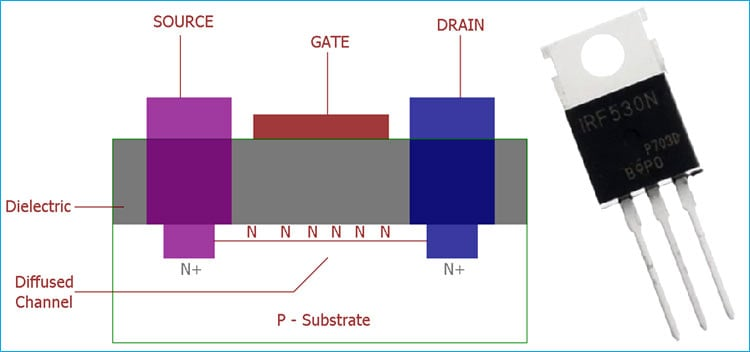MOSFET is basically a transistor which uses field effect. MOSFET stands for Metal Oxide Field Effect Transistor, which has a gate. The gate voltage determines the conductivity of the device. Depending on this gate voltage we can change the conductivity and thus we can use it as a switch or as an amplifier like we use Transistor as a switch or as an amplifier.

Bipolar Junction Transistor or BJT has base, emitter, and collector, whereas a MOSFET has gate, drain and source connection. Other than the pin configuration, BJT needs current for operation and MOSFET needs voltage.

MOSFET provides very high input impedance and it is very easy to bias. So, for a linear small amplifier, MOSFET is an excellent choice. The linear amplification occurs when we bias the MOSFET in the saturation region which is a centrally fixed Q point.

In the below image, a basic N-channel MOSFETs internal construction is shown. The MOSFET has three connections Drain, Gate, and Source. There is no direct connection present between the gate and the channel. The gate electrode is electrically insulated and due to this reason, it is sometimes referred to as IGFET or Insulated Gate Field Effect Transistor.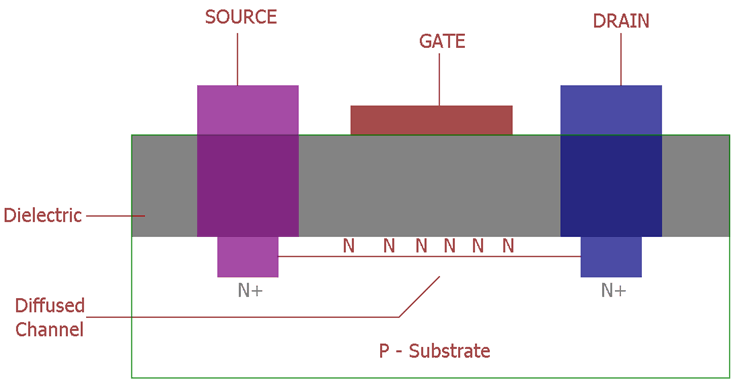Here is the image of widely popular MOSFET IRF530N.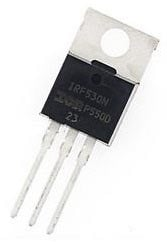### Types of MOSFETs

Based on the operating modes, there are two different types of MOSFETs available. These two types further have two subtypes

1. Depletion type MOSFET or MOSFET with Depletion mode
• N-Channel MOSFET or NMOS
• P-Channel MOSFET or PMOS
1. Enhancement type MOSFET or the MOSFET with Enhancement mode
• N-Channel MOSFET or NMOS
• P-Channel MOSFET or PMOS

Depletion type MOSFET

Depletion type of MOSFET is normally ON at zero Gate to Source voltage. If the MOSFET is N-Channel Depletion-type MOSFET then there will be some thresholds voltage, which is needed to make the device turn off. For example, an N-Channel Depletion MOSFET with a threshold voltage of -3V or -5V, the gate of the MOSFET needs to be pulled negative -3V or -5V to turn off the device. This threshold voltage will be Negative for the N channel, and positive in case of P channel. This type of MOSFET is generally used in logic circuits.

Enhancement type MOSFET

In Enhancement type of MOSFETs, the device remains OFF at zero Gate voltage. To turn on the MOSFET , we must provide a minimum Gate to Source voltage (Vgs Threshold voltage). But, the drain current is highly dependable on this gate-to-source voltage, if the Vgs is increased, the drain current also increases in the same manner. Enhancement type MOSFETs are ideal for constructing an Amplifier circuit. Also, similarly like depletion MOSFET, it also has the NMOS and PMOS subtypes.

### Characteristics and Curves of MOSFET

By providing the stable voltage across drain to source, we can understand the I-V curve of a MOSFET. As stated above, the drain current is highly dependable on the Vgs, gate to source voltage. If we vary the Vgs the Drain current will also vary.

Let's see the I-V curve of a MOSFET.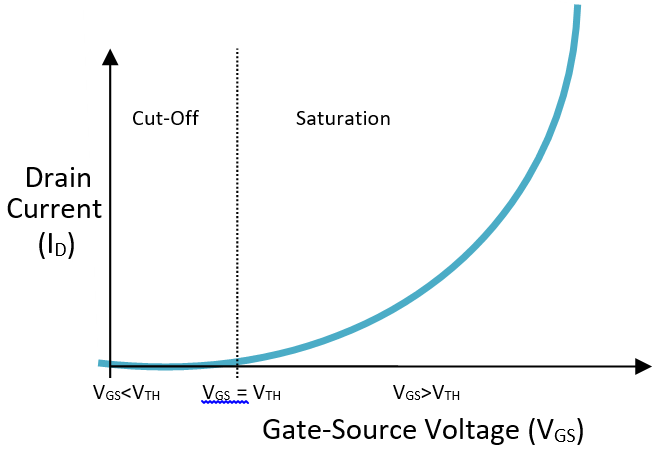In the above image, we can see the I-V slope of an N-Channel MOSFET, the drain current is 0 when the Vgs voltage is below the threshold voltage, during this time the MOSFET is in the cut-off mode. After that when gate-to-source voltage start increasing, the drain current also increases.

Let's see a practical example of IRF530 MOSFET’s I-V Curve,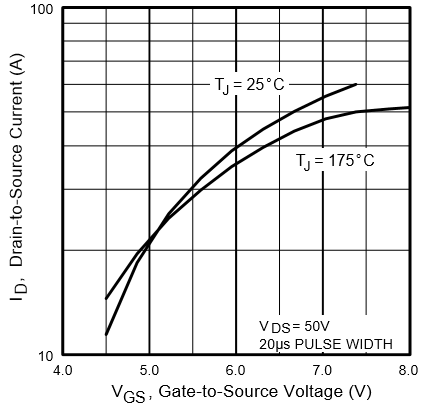The curve showing that when the Vgs is 4.5V, the maximum drain current of IRF530 is 1A at 25 degree C. But when we increase the Vgs to 5V, the Drain current is almost 2A, and finally at 6V Vgs, it can provide 10A of Drain Current.

### DC Biasing of MOSFET and Common-Source Amplification

Well, now it is the time to use a MOSFET as a linear Amplifier. It is not a tough job if we determine how to bias the MOSFET and use it in a perfect operation region.

MOSFET work in three operation modes: Ohmic, Saturation and Pinch off point. The saturation region also called as Linear Region. Here we operate the MOSFET in saturation region, it provides perfect Q-point.

If we provide a small signal (time-varying) and apply the DC bias at gate or input, then under the right situation the MOSFET provides linear amplification.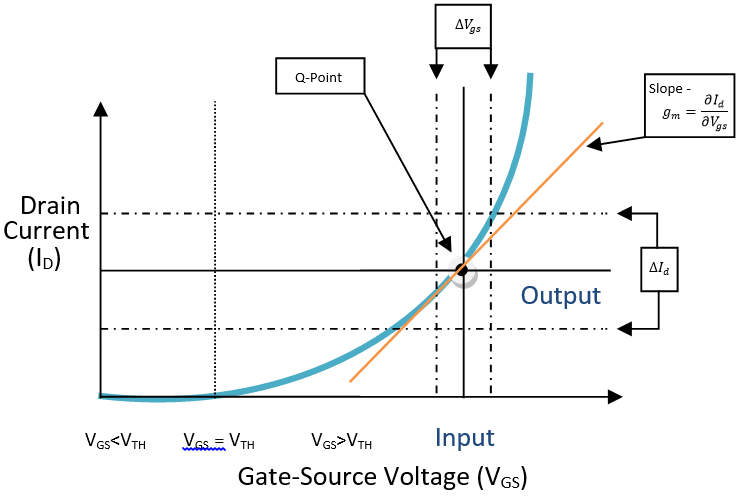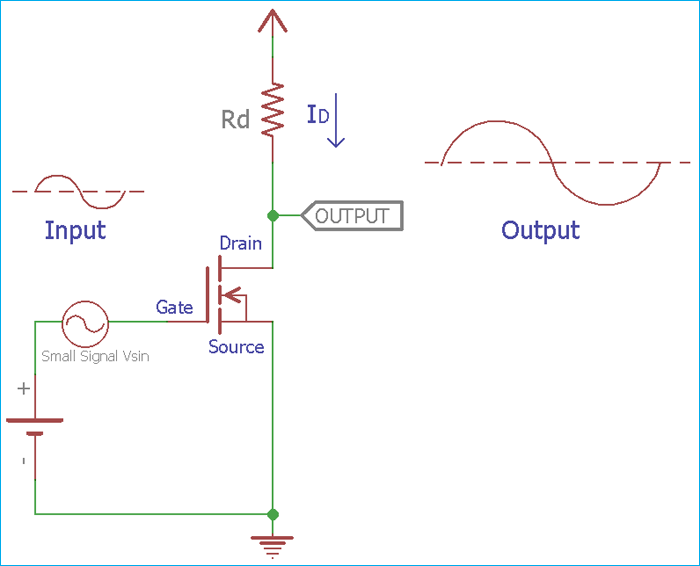In the above image, a small sinusoidal signal ( Vgs) is applied to the MOSFET gate, resulting in a fluctuation of drain current synchronous to the applied sinusoidal input. For the small signal Vgs, We can draw a straight line from the Q point which has a slope of gm = dId / dVgs.

The slope can be seen in the above image. This is the transconductance slope. It is an important parameter for the amplification factor. At this point the drain current amplitude is

`ߡId = gm x ߡVgs`

Now, if we look at the schematic given above, the drain resistor Rd can control the drain current as well as the drain voltage using the equation

`Vds = Vdd - Id x Rd (as V = I x R)`

AC output signal will be ߡVds = -ߡId x Rd = -gm x ߡVgs x Rd

Now by the equations, the gain will be

`Amplified Voltage Gain = -gm x Rd`

So, the overall gain of the MOSFET Amplifier is highly dependable on the transconductance and the Drain resistor.

### Basic Common Source Amplifier Construction with single MOSFET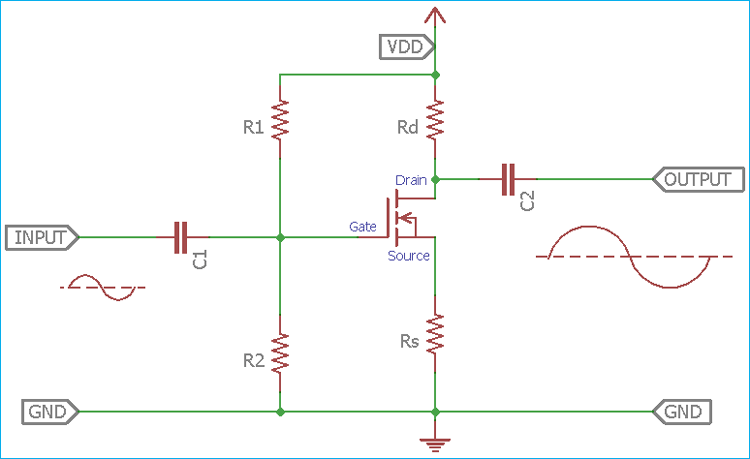To make a simple common source Amplifier using N channel single MOSFET, the important thing is to achieve DC biasing condition. To serve the purpose, a generic voltage divider is constructed using two simple resistors:  R1 and R2. Two more resistors are also required as Drain resistor and Source resistor.

To determine the value we need step by step calculation.

A MOSFET is provided with high input impedance, thus in operating condition, there is no current flow present in the gate terminal.

Now, if we look into the device, we will find that there are three resistors associated with VDD (Without the biasing resistors). The three resistors are Rd, MOSFET’s internal resistance and Rs. So, if we apply Kirchoff’s Voltage law then the voltages across those three resistors are equal to the VDD.

Now as per the Ohms law, if we multiply the current with resistor we will get voltage as V = I x R. So, here the current is Drain current or ID. Thus, the voltage across Rd is V = ID x Rd, same applies for the Rs as the current is the same ID, so the Voltage across Rs is Vs = ID x Rs. For the MOSFET, the Voltage is VDS or Drain-to-source voltage.

Now as per the KVL,

```VDD = ID x Rd + VDS + ID x Rs
VDD = ID (Rd + Rs) + VDS
(Rd + Rs) = VDD – VDS / ID  ```

We can further evaluate it as

```Rd = (VDD – VDS / ID)  - RS
Rs can caluculated as Rs = VS / ID  ```

Other two resistors values can be determined by the formula VG = VDD (R2 / R1 +R2)

If you do not have the  value, you can get it from the formula VG = VGS + VS

Fortunately, maximum values can be available from the MOSFET datasheet. Based on the specification we can build the circuit.

Two coupling capacitors are used to compensating the cut-off frequencies and to block the DC coming from the input or getting to the final output. We can simply get the values by finding out the equivalent resistance of the DC bias divider and then selecting the desired cutoff frequency. The formula will be

`C = 1 / 2πf Requirement`

For High power Amplifier design, we previously build a Power amplifier of 50 Watt using Two MOSFET as Push-pull configuration, follow the link for practical application.